## 4.1 Plasma diagnostics

The derived electron temperatures (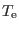) and densities (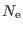) are listed in Table 5, together with the ionization potential required to create the emitting ions. We obtainedandfrom temperature-sensitive and density-sensitive emission lines by solving the equilibrium equations of level populations for a multilevel atomic model using EQUIB code (Howarth & Adams, 1981). The atomic data sets used for our plasma diagnostics from collisionally excited lines (CELs), as well as for abundances derived from CELs, are given in Table 4. The diagnostics procedure to determine temperatures and densities from CELs is as follows: we assume a representative initial electron temperature of 10000K in order to derivefrom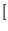II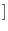line ratio; thenis derived fromIIline ratio in conjunction with the mean density derived from the previous step. The calculations are iterated to give self-consistent results forand. The correct choice of electron density and temperature is important for the abundance determination.

We see that the PN Abell 48 has a mean temperature of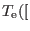IIK, and a mean electron density of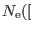II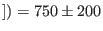cm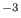, which are in reasonable agreement withII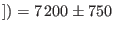K andII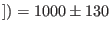cmfound by Todt et al. (2013). The uncertainty onII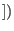is order ofpercent or more, due to the weak flux intensity of [N II]5755, the recombination contribution, and high interstellar extinction. Therefore, we adopted the mean electron temperature from our photoionization model for our CEL abundance analysis.

 Ion Transition probabilities Collision strengths N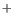Bell et al. (1995) Stafford et al. (1994) OZeippen (1987) Pradhan et al. (2006) O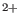Storey & Zeippen (2000) Lennon & Burke (1994) NeLandi & Bhatia (2005) McLaughlin & Bell (2000) SMendoza & Zeippen (1982) Ramsbottom et al. (1996) SMendoza & Zeippen (1982) Tayal & Gupta (1999) Huang (1985) ArBiémont & Hansen (1986) Galavis et al. (1995) Ion Recombination coefficient Case HStorey & Hummer (1995) B HePorter et al. (2013) B CDavey et al. (2000) B

Table 5 also lists the derived HeI temperatures, which are lower than the CEL temperatures, known as the ORL-CEL temperature discrepancy problem in PNe (see e.g. Liu et al., 2000; Liu et al., 2004b). To determine the electron temperature from the HeI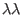5876, 6678 and 7281 lines, we used the emissivities of He I lines by Smits (1996), which also include the temperature range of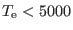K. We derived electron temperatures of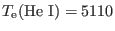K and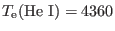K from the flux ratio HeI7281/5876 and7281/6678, respectively. Similarly, we got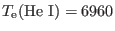K for HeI7281/5876 andK for7281/6678 from the measured nebular spectrum by Todt et al. (2013).

 Ion Diagnostic I.P.(eV)Ref.N II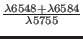14.53D13T13O III35.12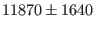T13 HeI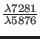24.59D13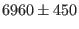T13 HeI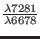24.59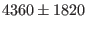D13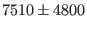T13S II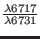10.36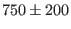D13T13

Ashkbiz Danehkar
2018-03-26